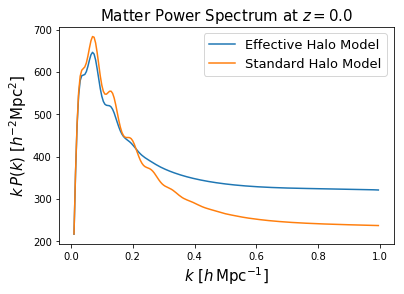# EffectiveHalos: Accurate Models for Matter Power Spectra and Cluster Covariances¶

EffectiveHalos is a fast Python code providing models of the real-space matter power spectrum, based a combination of the Halo Model and Effective Field Theory, which are 1% accurate up to $$k = 1h\,\mathrm{Mpc}^{-1}$$, across a range of cosmologies including those with massive neutrinos. It can additionally compute accurate halo count covariances (including a model of halo exclusion), both and alone and with the matter power spectrum, featuring a model for halo exclusion.

This is based on the work of [Philcox, Spergel & Villaescusa-Navarro 2020](https://arxiv.org/abs/2004.09515), and makes use of the CLASS and FAST-PT codes for computing linear and one-loop power spectra respectively.

Authors:

• Oliver Philcox (Princeton)
• David Spergel (Princeton / CCA)
• Francesco Villaescusa-Navarro (Princeton / CCA)

# Usage¶

To compute a matter power spectrum in EffectiveHalos, simply run the following:

from EffectiveHalos import *
import numpy as np

## Parameters
z = 0. # redshift
cs2 = 8. # effective speed of sound (should be calibrated from simulations)
R = 1. # smoothing scale (should be calibrated from simulations)
k = np.arange(0.01, 1., 0.005) # wavenumbers in h/Mpc

## Load general classes
cosmology = Cosmology(z, 'Planck18') # use Planck 2018 cosmology
mass_function = MassFunction(cosmology, 'Bhattacharya') # Bhattacharya 2010 mass function
halo_physics = HaloPhysics(cosmology, mass_function, 'Duffy', 'NFW') # Duffy 08 concentration relation, NFW halo profiles

## Load HaloModel class
halo_model = HaloModel(cosmology, mass_function, halo_physics, k)

## Compute the power spectrum in both Effective and Standard Halo Models
power_spectrum_EHM = halo_model.halo_model(cs2, R)
power_spectrum_SHM = halo_model.halo_model(cs2, R, 'Linear', 0, 0, 0)


This generates an estimate for the matter power spectrum in a few seconds. Let’s plot this:A full tutorial is given on the tutorial page.